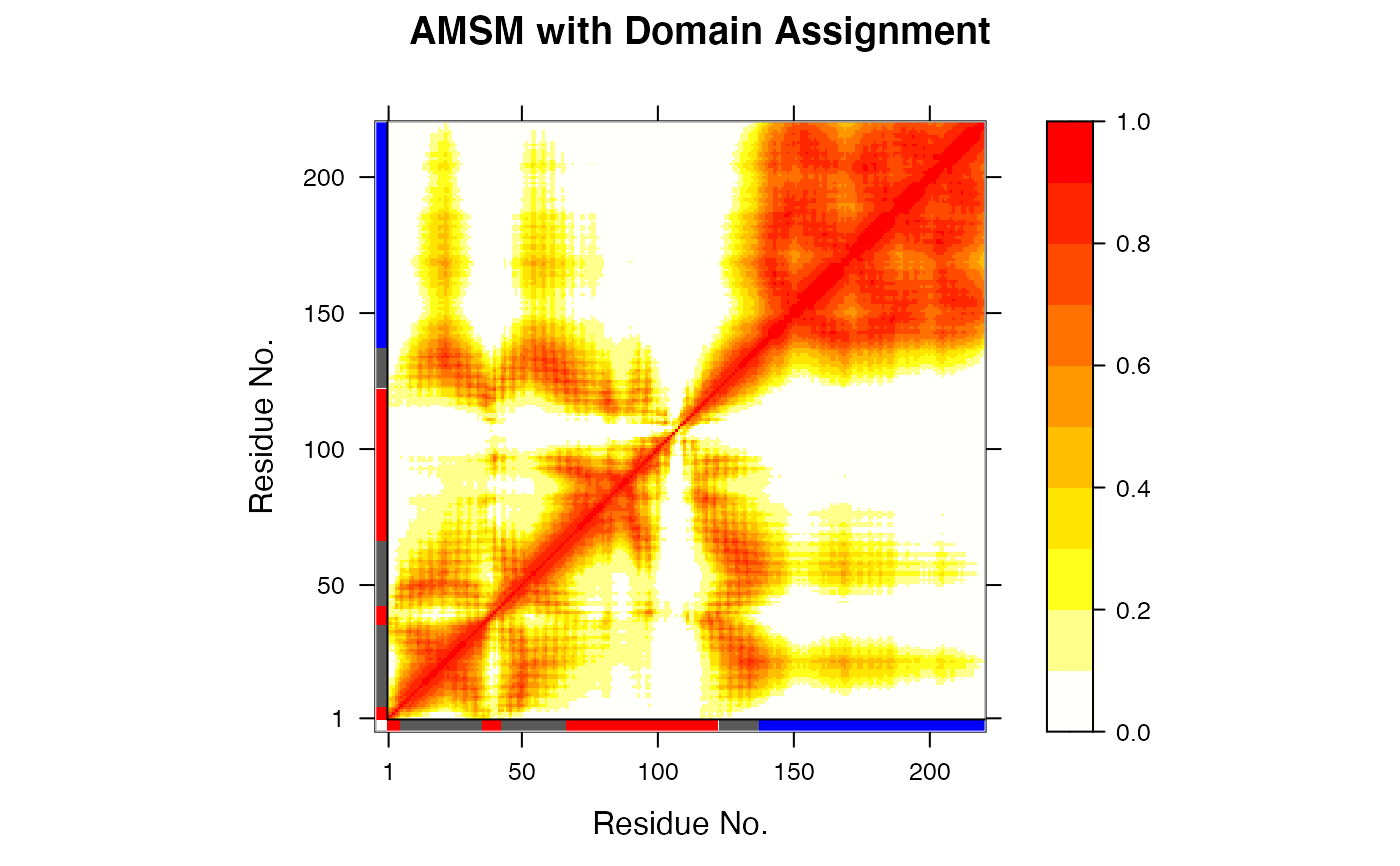Identifies geometrically stable domains in biomolecules

geostas(...)

# S3 method for default
geostas(...)

# S3 method for xyz
geostas(xyz, amsm = NULL, k = 3, pairwise = TRUE,
clustalg = "kmeans", fit = TRUE, ncore = NULL, verbose=TRUE, ...)

# S3 method for nma
geostas(nma, m.inds = 7:11, verbose=TRUE, ...)

# S3 method for enma
geostas(enma, pdbs = NULL, m.inds = 1:5, verbose=TRUE, ...)

# S3 method for pdb
geostas(pdb, inds = NULL, verbose=TRUE, ...)

# S3 method for pdbs
geostas(pdbs, verbose=TRUE, ...)

amsm.xyz(xyz, ncore = NULL)

# S3 method for geostas
print(x, ...)

## Arguments

... arguments passed to and from functions, such as kmeans, and hclust which are called internally in geostas.xyz. numeric matrix of xyz coordinates as obtained e.g. by read.ncdf, read.dcd, or mktrj. a numeric matrix as obtained by amsm.xyz (convenient e.g. for re-doing only the clustering analysis of the ‘AMSM’ matrix). an integer scalar or vector with the desired number of groups. logical, if TRUE use pairwise clustering of the atomic movement similarity matrix (AMSM), else columnwise. a character string specifing the clustering algorithm. Allowed values are ‘kmeans’ and ‘hclust’. logical, if TRUE coordinate superposition on identified core atoms is performed prior to the calculation of the AMS matrix. number of CPU cores used to do the calculation. ncore>1 requires package ‘parallel’ installed. logical, if TRUE details of the geostas calculations are printed to screen. an ‘nma’ object as obtained from function nma. Function mktrj is used internally to generate a trajectory based on the normal modes. the mode number(s) along which trajectory should be made (see function mktrj). an ‘enma’ object as obtained from function nma.pdbs. Function mktrj is used internally to generate a trajectory based on the normal modes. a ‘pdbs’ object as obtained from function pdbaln or read.fasta.pdb. a ‘pdb’ object as obtained from function read.pdb. a ‘select’ object as obtained from function atom.select giving the atomic indices at which the calculation should be based. By default the function will attempt to locate C-alpha atoms using function atom.select. a ‘geostas’ object as obtained from function geostas.

## Details

This function attempts to identify rigid domains in a protein (or nucleic acid) structure based on an structural ensemble, e.g. obtained from NMR experiments, molecular dynamics simulations, or normal mode analysis.

The algorithm is based on a geometric approach for comparing pairwise traces of atomic motion and the search for their best superposition using a quaternion representation of rotation. The result is stored in a NxN atomic movement similarity matrix (AMSM) describing the correspondence between all pairs of atom motion. Rigid domains are obtained by clustering the elements of the AMS matrix (pairwise=TRUE), or alternatively, the columns similarity (pairwise=FALSE), using either K-means (kmeans) or hierarchical (hclust) clustering.

Compared to the conventional cross-correlation matrix (see function dccm) the “geostas” approach provide functionality to also detect domains involved in rotational motions (i.e. two atoms located on opposite sides of a rotating domain will appear as anti-correlated in the cross-correlation matrix, but should obtain a high similarity coefficient in the AMS matrix).

See examples for more details.

## Note

The current implementation in Bio3D uses a different fitting and clustering approach than the original Java implementation. The results will therefore differ.

## Value

Returns a list object of type ‘geostas’ with the following components:

amsm

a numeric matrix of atomic movement similarity (AMSM).

fit.inds

a numeric vector of xyz indices used for fitting.

grps

a numeric vector containing the domain assignment per residue.

atomgrps

a numeric vector containing the domain assignment per atom (only provided for geostas.pdb).

inds

a list of atom ‘select’ objects with indices to corresponding to the identified domains.

Romanowska, J. et al. (2012) JCTC 8, 2588--2599. Skjaerven, L. et al. (2014) BMC Bioinformatics 15, 399. Grant, B.J. et al. (2006) Bioinformatics 22, 2695--2696.

## Author

Julia Romanowska and Lars Skjaerven

plot.geostas, read.pdb, mktrj, read.ncdf, read.dcd, nma, dccm.

## Examples

# \donttest{
# PDB server connection required - testing excluded

#### NMR-ensemble example
## Read a multi-model PDB file
#>   Note: Accessing on-line PDB file
## Find domains and write PDB
gs  <- geostas(pdb, fit=TRUE)
#>   .. 220 'calpha' atoms selected
#>   .. 'xyz' coordinate data with 20 frames
#>   .. 'fit=TRUE': running function 'core.find'
#>   .. coordinates are superimposed to core region
#>   .. calculating atomic movement similarity matrix ('amsm.xyz()')
#>   .. dimensions of AMSM are 220x220
#>   .. clustering AMSM using 'kmeans'
#>   .. converting indices to match input 'pdb' object
## Plot a atomic movement similarity matrix
plot.geostas(gs, contour=FALSE)## Fit all frames to the 'first' domain
domain.inds <- gs$inds[] xyz <- pdbfit(pdb, inds=domain.inds) #write.pdb(pdb, xyz=xyz, chain=gs$atomgrps)

# }

if (FALSE) {
#### NMA example
## Fetch stucture

## Calculate (vibrational) normal modes
modes <- nma(pdb)

## Find domains
gs <- geostas(modes, k=2)

## Write NMA trajectory with domain assignment
mktrj(modes, mode=7, chain=gs$grps) ## Redo geostas domain clustering gs <- geostas(modes, amsm=gs$amsm, k=5)

#### Trajectory example
## Read inn DCD trajectory file, fit coordinates
dcdfile <- system.file("examples/hivp.dcd", package = "bio3d")
xyz <- fit.xyz(trj[1,], trj)

## Find domains
gs <- geostas(xyz, k=3, fit=FALSE)

## Principal component analysis
pc.md <- pca.xyz(xyz)

## Visualize PCs with colored domains (chain ID)
mktrj(pc.md, pc=1, chain=gs$grps) #### X-ray ensemble GroEL subunits # Define the ensemble PDB-ids ids <- c("1sx4_[A,B,H,I]", "1xck_[A-B]", "1sx3_[A-B]", "4ab3_[A-B]") # Download and split PDBs by chain ID raw.files <- get.pdb(ids, path = "raw_pdbs", gzip = TRUE) files <- pdbsplit(raw.files, ids, path = "raw_pdbs/split_chain/") # Align structures pdbs <- pdbaln(files) # Find domains gs <- geostas(pdbs, k=4, fit=TRUE) # Superimpose to core region pdbs$xyz <- pdbfit(pdbs, inds=gs$fit.inds) # Principal component analysis pc.xray <- pca(pdbs) # Visualize PCs with colored domains (chain ID) mktrj(pc.xray, pc=1, chain=gs$grps)

##- Same, but more manual approach
gaps.pos <- gap.inspect(pdbs$xyz) # Find core region core <- core.find(pdbs) # Fit to core region xyz <- fit.xyz(pdbs$xyz[1, gaps.pos$f.inds], pdbs$xyz[, gaps.pos$f.inds], fixed.inds=core$xyz,
mobile.inds=core$xyz) # Find domains gs <- geostas(xyz, k=4, fit=FALSE) # Perform PCA pc.xray <- pca.xyz(xyz) # Make trajectory mktrj(pc.xray, pc=1, chain=gs$grps)

}